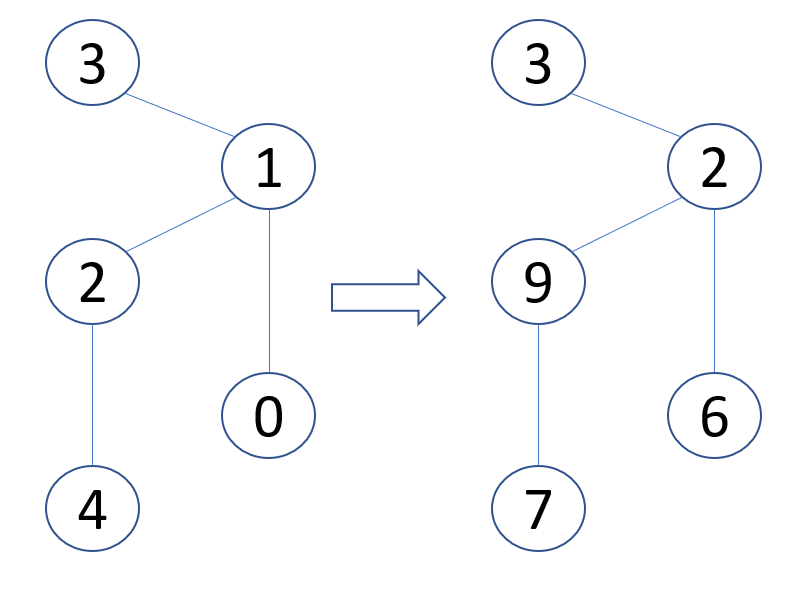# #569. 【IOI2020】Stations

1. 执行路由函数，找到 $z$ 到 $y$ 的唯一路径中 $z$ 的邻居。然后
2. 将数据包转发给这个邻居。

• 第一个函数的输入参数为 $n$、主干网链路的列表和一个整数 $k \ge n - 1$。该函数需要为每个站点分配一个独一无二的编号，其大小在 $0$ 到 $k$ 之间（包括 $0$ 和 $k$）。
• 第二个函数是路由函数，它在站点编号分配好后部署到所有站点上。
• 它的输入参数如下：
• $s$，数据包当前所处的站点的编号
• $t$，数据包的目的站点的编号（$t \ne s$），
• $c$，表示 $s$ 的所有邻居站点的编号的列表。
• 该函数应该返回一个 $s$ 的邻居的编号，表示数据包需要转发到的下个站点。

### 实现细节

int[] label(int n, int k, int[] u, int[] v)
• $n$：主干网中站点的数量。
• $k$：可用的编号的最大值。
• $u$ 和 $v$：大小为 $n - 1$ 的数组，表示链路。对每个 $i$（$0 \le i \le n - 2$），链路 $i$ 连接着序号为 $u[i]$ 和 $v[i]$ 的站点。
• 该函数应该返回一个大小为 $n$ 的数组 $L$。对每个 $i$（$0 \le i \le n-1$），$L[i]$ 表示序号为 $i$ 的站点所分配到的编号。数组 $L$ 中的所有元素必须互不相同并且大小在 $0$ 到 $k$ 之间。
int find_next_station(int s, int t, int[] c)
• $s$：数据包当前所在站点的编号。
• $t$：数据包目的站点的编号。
• $c$：一个数组，包含 $s$ 的所有邻居的编号。数组 $c$ 按照元素大小升序排列。
• 该函数应该返回一个 $s$ 的邻居的编号，表示数据包需要转发到的下个站点。

• label 函数被调用 $r$ 次，

• 返回的编号将被评测系统保存，并且

• find_next_station 不会被调用。

• find_next_station 会被调用若干次。对于每次调用，评测程序会选择任意某个场景，该场景中的 label 函数所返回的编号方式将用于本次 find_next_station 调用。

• label 不会被调用。

### 例子

label(5, 10, [0, 1, 1, 2], [1, 2, 3, 4])

0 6
1 2
2 9
3 3
4 7find_next_station(9, 6, [2, 7])

find_next_station(2, 3, [3, 6, 9])

### 约束条件

• $1 \leq r \leq 10$

• $2 \leq n \leq 1000$
• $k \geq n-1$
• $0 \leq u[i], v[i] \leq n-1$ （对于所有 $0 \leq i \leq n-2$）

• $s$ 和 $t$ 是两个不同站点的编号。

• $c$ 是编号为 $s$ 的站点的所有邻居的编号的序列，升序排列。

### 子任务

1. （5分）$k=1000$，不会出现拥有多于 $2$ 个邻居的站点。
2. （8分）$k=1000$，链路 $i$ 连接站点 $i+1$ 和 $\left\lfloor\frac{i}{2}\right\rfloor$。
3. （16分）$k=1000000$，最多一个站点拥有多于 $2$ 个的邻居。
4. （10分）$n \leq 8, k=10^{9}$
5. （61分）$k=10^{9}$

$m \geq 10^9$ $0$
$2000\leq m \lt 10^9$ $50 \cdot \log _{5 \cdot 10^{5}}\left(\frac{10^{9}}{m}\right)$
$1000\lt m \lt 2000$ $50$
$m \leq 1000$ $61$

### 评测程序示例

• 第 $1$ 行：$r$

• 第 $1$ 行：$n$ $k$
• 第 $2+i$（$0 \leq i \leq n-2$）行: $u[i] v[i]$
• 第 $1+n$ 行： $q$，find_next_station 的调用次数
• 第 $2+n+j$（$0 \leq j \leq q-1$）行: $z[j]$ $y[j]$ $w[j]$，第 $j$ 次调用 find_next_station 时所涉及的站点的序号。此时，数据包在站点 $z[j]$，目的站点为 $y[j]$，应该要转发给站点 $w[j]$。

• 第 $1$ 行：$m$

• 第 $1+j$（$0 \leq j \leq q-1$）行：站点的序号，它所对应的编号是第 $j$ 次调用 find_next_station 时返回的结果。

### hack

hack 时，数据格式与上述类似，但是您需要在最后额外添加一行两个数，第一个数为 0/1，表示是否给予部分分，第二个数为 $[0, 2^{31}-1]$ 内的整数，为打乱询问顺序的种子。